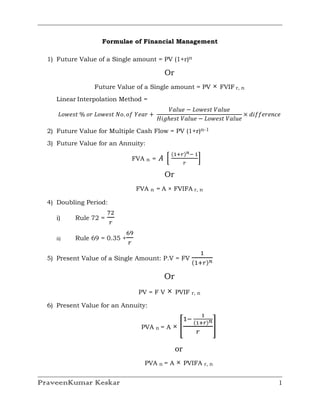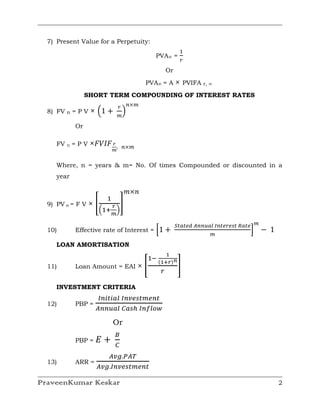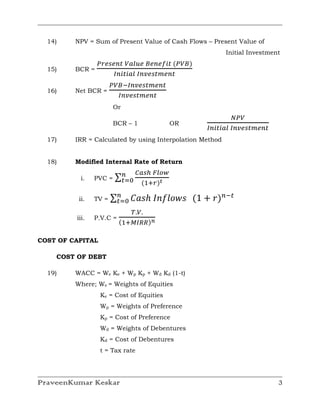Successfully reported this slideshow.

# Formulae of financial management

27

Share×
1 of 6
1 of 6

# Formulae of financial management

27

Share

## More Related Content

### Related Books

Free with a 14 day trial from Scribd

See all

### Related Audiobooks

Free with a 14 day trial from Scribd

See all

### Formulae of financial management

1. 1. Formulae of Financial Management 1) Future Value of a Single amount = PV (1+r)n Or Future Value of a Single amount = PV × FVIF r, n Linear Interpolation Method = ܸ݈ܽ‫݁ݑ݈ܸܽ ݐݏ݁ݓ݋ܮ − ݁ݑ‬ ‫ + ݎܻܽ݁ ݂݋ .݋ܰ ݐݏ݁ݓ݋ܮ ݎ݋ % ݐݏ݁ݓ݋ܮ‬ × ݂݂݀݅݁‫݁ܿ݊݁ݎ‬ ‫݃݅ܪ‬ℎ݁‫݁ݑ݈ܸܽ ݐݏ݁ݓ݋ܮ − ݁ݑ݈ܸܽ ݐݏ‬ 2) Future Value for Multiple Cash Flow = PV (1+r)n-1 3) Future Value for an Annuity: ሺଵା௥ሻ೙ ି ଵ ‫ ܣ‬ቂ ቃ ௥ FVA n = Or FVA n = A × FVIFA r, n 4) Doubling Period: ଻ଶ ௥ i) Rule 72 = ଺ଽ ௥ ii) Rule 69 = 0.35 + ଵ ሺଵା௥ሻ೙ 5) Present Value of a Single Amount: P.V = FV Or PV = F V × PVIF r, n 6) Present Value for an Annuity: భ ଵି ሺభశೝሻ೙ ×ቈ ቉ ௥ PVA n =A or PVA n =A × PVIFA r, n PraveenKumar Keskar 1
2. 2. 7) Present Value for a Perpetuity: ଵ ௥ PVA∞ = Or PVA∞ = A × PVIFA r, ∞ SHORT TERM COMPOUNDING OF INTEREST RATES ௥ ௡×௠ × ቀ1 + ቁ ௠ 8) FV n =PV Or FV n =PV ×‫ ܨܫܸܨ‬ೝ , ௡×௠ ೘ Where, n = years & m= No. Of times Compounded or discounted in a year ௠×௡ ଵ ×ቈ ೝ ቉ ቀଵା ቁ 9) PV n = F V ೘ ௌ௧௔௧௘ௗ ஺௡௡௨௔௟ ூ௡௧௘௥௘௦௧ ோ௔௧௘ ௠ ቂ1 + ቃ − 1 ௠ 10) Effective rate of Interest = LOAN AMORTISATION భ ଵି ሺభశೝሻ೙ ×ቈ ቉ ௥ 11) Loan Amount = EAI INVESTMENT CRITERIA ூ௡௜௧௜௔௟ ூ௡௩௘௦௧௠௘௡௧ ஺௡௡௨௔௟ ஼௔௦௛ ூ௡௙௟௢௪ 12) PBP = Or ஻ PBP = ‫ + ܧ‬஼ ஺௩௚.௉஺் ஺௩௚.ூ௡௩௘௦௧௠௘௡௧ 13) ARR = PraveenKumar Keskar 2
3. 3. 14) NPV = Sum of Present Value of Cash Flows – Present Value of Initial Investment ௉௥௘௦௘௡௧ ௏௔௟௨௘ ஻௘௡௘௙௜௧ ሺ௉௏஻ሻ ூ௡௜௧௜௔௟ ூ௡௩௘௦௧௠௘௡௧ 15) BCR = ௉௏஻ିூ௡௩௘௦௧௠௘௡௧ ூ௡௩௘௦௧௠௘௡௧ 16) Net BCR = Or ே௉௏ ூ௡௜௧௜௔௟ ூ௡௩௘௦௧௠௘௡௧ BCR – 1 OR 17) IRR = Calculated by using Interpolation Method 18) Modified Internal Rate of Return ஼௔௦௛ ி௟௢௪ ∑௡ ௧ୀ଴ ሺଵା௥ሻ೟ i. PVC = ii. TV = ∑௡ ‫ݏܽܥ‬ℎ ‫ ݏݓ݋݈݂݊ܫ‬ሺ1 + ‫ݎ‬ሻ௡ି௧ ௧ୀ଴ ்.௏. ሺଵାெூோோሻ೙ iii. P.V.C = COST OF CAPITAL COST OF DEBT 19) WACC = We Ke + Wp Kp + Wd Kd (1-t) Where; We = Weights of Equities Ke = Cost of Equities Wp = Weights of Preference Kp = Cost of Preference Wd = Weights of Debentures Kd = Cost of Debentures t = Tax rate PraveenKumar Keskar 3
4. 4. 20) Irredeemable Debentures ூ ஼ெ௉ ௢௥ ே௉ a. Pre Tax Cost of Debt = Kd = Where, Kd = Cost of Capital I = Interest CMP = Current Market Price NP = Net Sales Proceeds ூ ሺூି௧ሻ b. Post Tax Cost of Debt = Kd = ஼ெ௉ ௢௥ ே௉ Where, t = Corporate Tax 21) Redeemable Debentures ሺಷషುబ ሻ ூା ೙ ଴.଺ሺ௉బ ሻା ଴.ସ ሺிሻ a. Pre Tax Cost of Debt = Kd = ಷషುబ ூ ሺଵି௧ ሻା ೙ ଴.଺ሺ௉బ ሻା ଴.ସ ሺி ሻ b. Post Tax Cost of Debt = Kd = 1. Approximation Formula: ሺಷషುబ ሻ ூା ೙ ଵ/ଶ ሺிା௉బ ሻ a. Pre Tax = Kd = ሺಷషುబ ሻ ூሺூା௧ሻା ೙ ଵ/ଶ ሺிା௉బ ሻ b. Post Tax = Kd = COST OF PREFERENCE CAPITAL 22) Irredeemable Preference Share ஽ ஼ெ௉ ௢௥ ே௉ a) Without dividend tax = Kp = ஽ ሺଵା௧ೣ ሻ ஼ெ௉ ௢௥ ே௉ b) With dividend tax = Kp = PraveenKumar Keskar 4
5. 5. 23) Redeemable Preference Share ሺಷషುሻ ஽ା ೙ ଴.଺ሺ௉ ሻା଴.ସሺிሻ a) Without dividend tax = Kp = ሺಷషುሻ ஽ ሺଵି஽೟ ሻା b) With dividend tax = Kp = ೙ ଴.଺ሺ௉ ሻା଴.ସሺிሻ COST OF TERM LOAN 24) Kt = R (1-t) COST OF EQUITY ஽భ ஼ெ௉ ௢௥ ே௉ 25) Dividend Capitalisation Approach= Ke = Where, D1 =Dividend/Share 26) Dividend Capitalisation + Growth Rate Approach = ஽భ + ݃ ஼ெ௉ ௢௥ ே௉ Ke = Where, ݃ = Growth rate 27) Capital Asset Pricing Model or Security Market Line: Ke = Rf + β (Rm - Rf) Where, Rf = Risk free rate of return. Rm = market return of portfolio β = Beta Rm - Rf = Market Risk Premium 28) Cost of Equity of Bond Yield Ke = Yield on Long term bonds + Risk Premium 29) Earnings Capitalisation Approach: ாభ ஼ெ௉ ௢௥ ே௉ Ke = Where, E1 = Expected Earnings/Share PraveenKumar Keskar 5
6. 6. 30) Cost of Retained Earnings: Kr = Ke CAPITAL STRUCTURE NET INCOME APPROACH ஽ ா 31) WACC = ቀ஽ାாቁ ‫ܭ‬ௗ + ቀ஽ାாቁ ‫ܭ‬௘ ூ௡௧௘௥௘௦௧ ௄೏ 32) D= ேைூିூ௡௧௘௥௘௦௧ ௄೐ 33) E= NET OPERATING INCOME APPROACH ஽ K ୢ = ‫ܭ‬ை ሺ‫ܭ‬௢ − ‫ܭ‬ௗ ሻሺ ሻ ா 34) ேைூ ௄ೀ 35) V= PraveenKumar Keskar 6